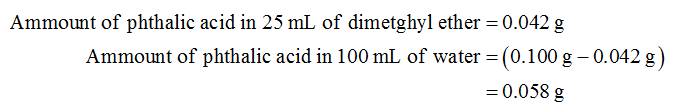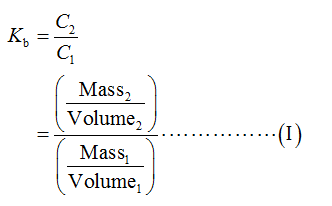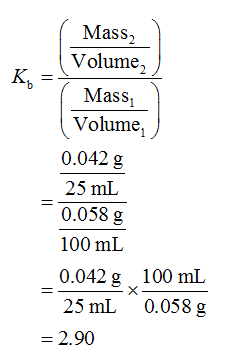# A 0.100 gram sample of phthalic acid was dissolved in 100 mL of water. When 25 of diethyl ether was used to extract the phthalic acid, 0.042 grams of phthalic acid re recovered. What is the distribution coefficient for this extraction Kp?

Question
25 views

A 0.100 gram sample of phthalic acid was dissolved in 100 mL of water. When 25 of diethyl ether was used to extract the phthalic acid, 0.042 grams of phthalic acid re recovered. What is the distribution coefficient for this extraction Kp?

check_circle

Step 1

Given data:Step 2

The distribiution coefficient for the given extraction is calculated as shown below.Step 3

Substitute the given data in equation (I) to calculate distribiution coefficient....

### Want to see the full answer?

See Solution

#### Want to see this answer and more?

Solutions are written by subject experts who are available 24/7. Questions are typically answered within 1 hour.*

See Solution
*Response times may vary by subject and question.
Tagged in

### Chemistry